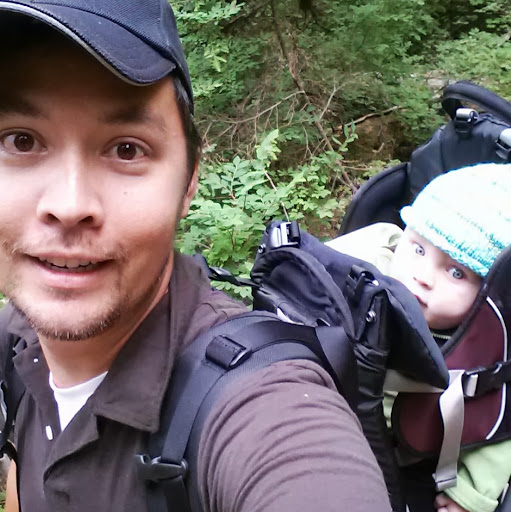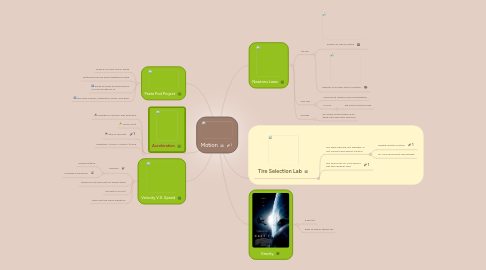# MotionDj Ellis

Solve your problems or get new ideas with basic brainstorming

Начать. Это бесплатно
или регистрация c помощью Вашего email-адресаMotion создатель## 1. Newtons Laws

### 1.1. 1st law

1.1.1. Known as law of Inertia

1.1.2. Objects in motion stay in motion.

### 1.2. 2nd law

1.2.1. More force means more acceleration.

1.2.2. F=m*a

1.2.2.1. we used a spring scale.

### 1.3. 3rd law

1.3.1. For every action there is an equal and opposite reaction.

## 3. Tire Selection Lab

### 3.1. We were figuring out whether or not surface area affect friction.

3.1.1. Weight affects friction

3.1.2. Mr. Ellis should just add weight.

## 4. Velocity V.S. Speed

### 4.1. Velocity

4.1.1. Distance/time

4.1.2. Includes a Direction